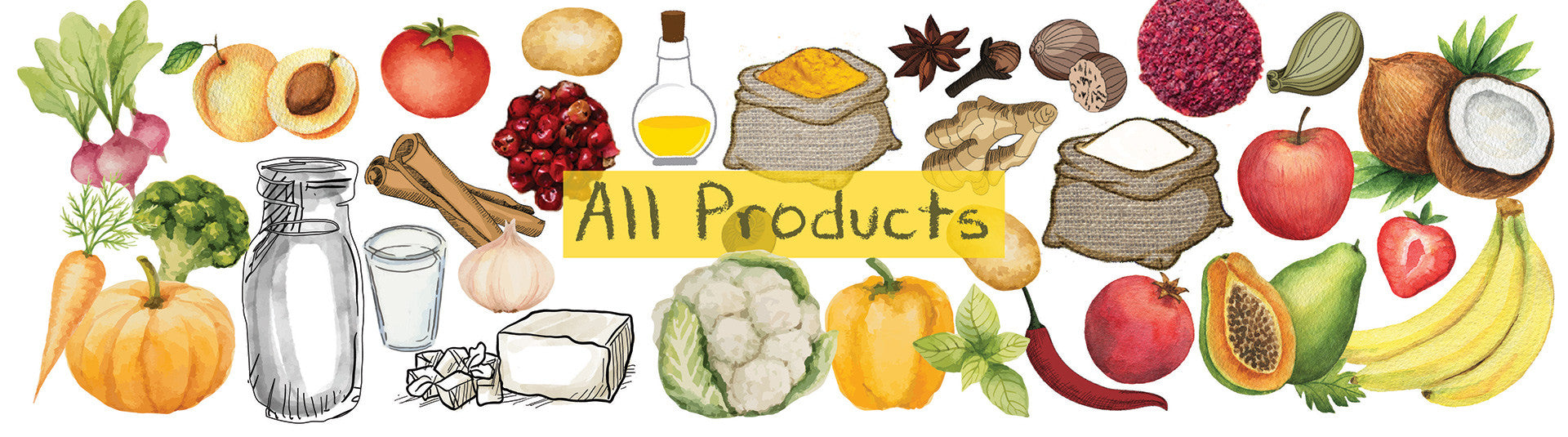Potato, Onion, Tomato (2Kg each) Combo
Unit
Yogis Pass Price: Rs. 590.00
Rs. 590.00
Apple
Unit
Yogis Pass Price: Rs. 175.50
Rs. 195.00
Banana
Unit
Yogis Pass Price: Rs. 43.20
Rs. 48.00
Kiwi
Unit
Yogis Pass Price: Rs. 117.00
Rs. 130.00
Mango-Alphonso
Unit
Yogis Pass Price: Rs. 438.75
Rs. 487.50
Mango-Dasheri
Unit
Yogis Pass Price: Rs. 175.50
Rs. 195.00
Mango-Kesar
Unit
Yogis Pass Price: Rs. 175.50
Rs. 195.00
Mango-Safeda
Unit
Yogis Pass Price: Rs. 168.75
Rs. 187.50
Papaya
Unit
Yogis Pass Price: Rs. 223.88
Rs. 248.75
Pineapple
Unit
Yogis Pass Price: Rs. 268.65
Rs. 298.50
Muskmelon
Unit
Yogis Pass Price: Rs. 247.50
Rs. 275.00
Pomegranate (Anaar)
Unit
Yogis Pass Price: Rs. 202.50
Rs. 225.00
Sapota (Chikoo) - Semi Ripe
Unit
Yogis Pass Price: Rs. 146.25
Rs. 162.50
Sweet Lime
Unit
Yogis Pass Price: Rs. 112.50
Rs. 125.00
Watermelon (Tarbooz)
Unit
Yogis Pass Price: Rs. 247.50
Rs. 275.00
Alocasia tubers (Arbi)
Unit
Yogis Pass Price: Rs. 36.00
Rs. 62.50
Baby Corn
Unit
Yogis Pass Price: Rs. 81.00
Rs. 90.00
Banana - Raw
Unit
Yogis Pass Price: Rs. 28.13
Rs. 31.25
Basil
Unit
Yogis Pass Price: Rs. 63.00
Rs. 70.00
Beans - French/String
Unit
Yogis Pass Price: Rs. 49.50
Rs. 55.00
Beetroot
Unit
Yogis Pass Price: Rs. 36.00
Rs. 40.00
Bitter Gourd (Karela)
Unit
Yogis Pass Price: Rs. 42.75
Rs. 47.50
Bottle Gourd (Ghia)
Unit
Yogis Pass Price: Rs. 58.50
Rs. 65.00
Brinjal Big
Unit
Yogis Pass Price: Rs. 31.50
Rs. 35.00
Brinjal Small
Unit
Yogis Pass Price: Rs. 29.25
Rs. 32.50
Broccoli
Unit
Yogis Pass Price: Rs. 179.64
Rs. 199.60
Cabbage
Unit
Yogis Pass Price: Rs. 63.00
Rs. 70.00
Capsicum
Unit
Yogis Pass Price: Rs. 42.75
Rs. 47.50
Carrot- Orange
Unit
Yogis Pass Price: Rs. 44.77
Rs. 49.75
Carrot (Red)
Unit
Yogis Pass Price: Rs. 44.77
Rs. 49.75
Cauliflower
Unit
Yogis Pass Price: Rs. 89.55
Rs. 99.50
Celery
Unit
Yogis Pass Price: Rs. 129.38
Rs. 143.75
Cherry Tomatoes
Unit
Yogis Pass Price: Rs. 123.75
Rs. 137.50
Choliya (Green Chana)
Unit
Yogis Pass Price: Rs. 269.55
Rs. 299.50
Coriander
Unit
Yogis Pass Price: Rs. 31.50
Rs. 35.00
Corn on the Cob/Sweet Corn
Unit
Yogis Pass Price: Rs. 67.50
Rs. 75.00
Cucumber
Unit
Yogis Pass Price: Rs. 29.25
Rs. 32.50
Cucumber - English/Seedless
Unit
Yogis Pass Price: Rs. 42.75
Rs. 47.50
Fenugreek leaves (Methi)
Unit
Yogis Pass Price: Rs. 51.75
Rs. 57.50
Garlic
Unit
Yogis Pass Price: Rs. 32.40
Rs. 36.00
Ginger
Unit
Yogis Pass Price: Rs. 30.60
Rs. 34.00
Green Chillies
Unit
Yogis Pass Price: Rs. 8.96
Rs. 9.95
Green Lettuce
Unit
Yogis Pass Price: Rs. 146.25
Rs. 162.50
Ice Berg Lettuce
Unit
Yogis Pass Price: Rs. 269.55
Rs. 299.50
Indian Gooseberry (Amla)
Unit
Yogis Pass Price: Rs. 78.75
Rs. 87.50
Jackfruit Raw - Cut Pieces
Unit
Yogis Pass Price: Rs. 146.25
Rs. 162.50
Unit
Yogis Pass Price: Rs. 44.77
Rs. 49.75
Leek
Unit
Yogis Pass Price: Rs. 112.50
Rs. 125.00
Lemon
Unit
Yogis Pass Price: Rs. 31.50
Rs. 35.00
Local Greens (Bathua)
Unit
Yogis Pass Price: Rs. 56.25
Rs. 62.50
Lotus Stem (Kamal Kakdi)
Unit
Yogis Pass Price: Rs. 67.50
Rs. 75.00
Mint
Unit
Yogis Pass Price: Rs. 10.00
Rs. 12.00
Mushroom
Unit
Yogis Pass Price: Rs. 108.00
Rs. 120.00
Mustard Greens (Sarson)
Unit
Yogis Pass Price: Rs. 67.50
Rs. 75.00
Onion
Unit
Yogis Pass Price: Rs. 49.50
Rs. 55.00
Parsley
Unit
Yogis Pass Price: Rs. 67.50
Rs. 75.00
Unit
Yogis Pass Price: Rs. 40.50
Rs. 45.00
Pepper Red
Unit
Yogis Pass Price: Rs. 126.00
Rs. 140.00
Pepper Yellow
Unit
Yogis Pass Price: Rs. 126.00
Rs. 140.00
Peas
Unit
Yogis Pass Price: Rs. 73.13
Rs. 81.25
Potato
Unit
Yogis Pass Price: Rs. 31.50
Rs. 35.00
Raw Papaya
Unit
Yogis Pass Price: Rs. 135.00
Rs. 150.00
Raw Turmeric
Unit
Yogis Pass Price: Rs. 22.50
Rs. 25.00
Sponge Gourd (Tori)
Unit
Yogis Pass Price: Rs. 50.63
Rs. 56.25
Spring Onion
Unit
Yogis Pass Price: Rs. 56.25
Rs. 62.50
Unit
Yogis Pass Price: Rs. 89.55
Rs. 99.50
Tomato - Hybrid
Unit
Yogis Pass Price: Rs. 63.00
Rs. 70.00
Tomato - Desi
Unit
Yogis Pass Price: Rs. 63.00
Rs. 70.00
Zucchini - Green
Unit
Yogis Pass Price: Rs. 84.38
Rs. 93.75
Zucchini - Yellow
Unit
Yogis Pass Price: Rs. 89.78
Rs. 99.75
Cottage Cheese (Paneer)
Unit
Yogis Pass Price: Rs. 134.78
Rs. 149.75
Almond Butter Smooth
Unit
Yogis Pass Price: Rs. 494.10
Rs. 549.00
Almond Butter Unsweetened
Unit
Yogis Pass Price: Rs. 494.10
Rs. 549.00
Peanut Butter Crunchy
Unit
Yogis Pass Price: Rs. 224.10
Rs. 249.00
Peanut Butter Crunchy Large
Unit
Yogis Pass Price: Rs. 494.10
Rs. 549.00
Peanut Butter Unsweetened
Unit
Yogis Pass Price: Rs. 224.10
Rs. 249.00
Peanut Butter Unsweetened Large
Unit
Yogis Pass Price: Rs. 494.10
Rs. 549.00
Wild Flower Honey
Unit
Yogis Pass Price: Rs. 224.10
Rs. 249.00
Almonds
Unit
Yogis Pass Price: Rs. 180.00
Rs. 200.00
Chia seeds
Unit
Yogis Pass Price: Rs. 112.50
Rs. 125.00
Flax Seeds
Unit
Yogis Pass Price: Rs. 45.00
Rs. 50.00
Sesame White (Til)
Unit
Yogis Pass Price: Rs. 58.50
Rs. 65.00
Quinoa
Unit
Yogis Pass Price: Rs. 179.10
Rs. 199.00
Black Pepper Powder
Unit
Yogis Pass Price: Rs. 166.50
Rs. 185.00
Carom Seeds (Ajwain)
Unit
Yogis Pass Price: Rs. 76.50
Rs. 85.00
Cinnamon Powder
Unit
Yogis Pass Price: Rs. 94.50
Rs. 105.00
Cinnamon Sticks
Unit
Yogis Pass Price: Rs. 90.00
Rs. 100.00